International Journal of Science, Technology and Society
Volume 3, Issue 3, May 2015, Pages: 96-100

Study Shapes and Alignments of Rotational States in Some Rare-Earth Nuclei

N. A. Mansour, N. M. Eldebawi

Faculty of Science, Physics Department, Zagazig University, Zagazig, Egypt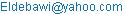(N. M. Eldebawi)

N. A. Mansour, N. M. Eldebawi. Study Shapes and Alignments of Rotational States in Some Rare-Earth Nuclei. International Journal of Science, Technology and Society. Vol. 3, No. 3, 2015, pp. 96-100

Abstract: The ground state rotational band members for the studied nuclei have been identified and their energies calculated to about ±0.3%. These energies provide good test of various models for rotational bands, and impressive agreement is observed with a theoretical calculations by the variable moment of inertia (VMI) model and cubic polynomial (CP) formula. In addition to giving an excellent fit to the excitation energy of the ground-band levels in all nuclei. Also reproduces a critical spin Ic beyond which the square rotational frequency ω2 decreases although the moment of inertia keeps on increasing, thus resulting in back bending in-ω2 plot for some of the studied nuclei. This feature is predicted to appear not only in the deformed nuclei but also at quite low spins in nearly spherical nuclei as well. Also the formula contains the low and high spin behavior in agreement with the available experimental data.

Keywords: Rotational Parameter, Moment of Inertia, Back-Bending

1. Introduction

The study of nuclear states has in recent years expanded to include still higher angular momenta and excitation energies (1-3) by an interplay between deformation and alignment effects. Theoretical calculations predict various shapes: some are prolate or triaxial, others (near closed shells) become oblate for a certain spin interval. But at the highest spins the nuclei have a tendency towards a large triaxial deformation and sometimes towards very large prolate deformations (super deformations). Directly involved in their shape changes (3) are aligned orbitals which come down to the Fermi level as the nucleus rotates more rapidly. At a certain frequency these orbitals become populated and cause large alignments. These some orbitals also come down in energy as the deformation increases and therefore constitute a driving force towards large deformations. Moments of inertia are sensitive to shapes and alignments. In the present paper, I shall focus on a moment of inertia, which is proportional to the height of angular momentum obtained in the de-excitation of compound nuclei; the moment of inertia is a smooth and single-valued function of the rotational frequency. The nuclides 154Sm92 (6) , 158Gd94 (7,8), 162Dy96 (9,10), 164,166Er98 (11,12) , 168Yb100 (13), 174Hf102 (14,15) 178W104 (16) and 182Os106 (17,18,19,20,21,and 22) have been chosen for the present work as an illustration out of an extensive investigation concerning the behavior of the ground state rotational bands in doubly even nuclides. These nuclides have been chosen because they show a quite different behavior of the band structures near the top of the bands and any theoretical description will ultimately have to explain these differences. The nuclei studied exhibits a behavior similar to the one 168Yb, i-e, the moment of inertia is a smooth and single-valued function of the rotational frequency (5) .

2. Description of the Models and the Formalism in the Analysis

Our procedure from adjusting the excitation energies of partially level schemes for the studied nuclei is discussed in more general terms. In the absence of detailed microscopic calculations attempts have been made to describe the dependence of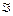on I (or ω2) phenomenological. The analysis is based on a variational expression for the energy (using VMI model).

EI = [I (I+1)/2] + V ()            (1)

V () = c (-0)               (2)

where c and0 are parameters characteristic for a given nucleus.(I) is determined by the requirement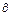E /| I = 0               (3)

were shown to describe with surprising accuracy the energies of yarest bands in even-even nuclei (I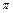= 0+ , 2+ , 4+ , 6+ , 8+ ,….,up to Ic ,the critical angular momentum at which a sudden increase ofoccurs [back bending behavior Ref. (23) has appeared in Fig. (6)]). The limit of validity of the equations 1, 2 and 3 is reached for0 = -, giving EI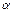[I (I + 1)] ½ . Between(0) = 0 and(0) =, the ground state moment of inertia is(0) = 0 . The extension of equations 1, 2 and 3 to(0)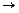-permitted the definition of the average moment of inertia02 = ½ [(0)+(2)] , which laid the basis for a macroscopic description of the effective moment of inertia . As0 reaches larger and larger negative values, the nuclear resistance to cranking increases until, at0 = -, the threshold energy ½ c (0 )2 diverges (24) . The coefficients c has been determined by a least-squares fit weighted by the inverse square of the measured energy, that is, minimize the relative errors. The components of the rotational frequency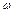and the moment of inertiaI are deduced from the data by defining

ħω = E ( II-2 ) / I(I+1)½ - [(I-1)(I-2)]½    (4)

and

2I / ħ2 = (4I-2) / E ( II-2 )     (5)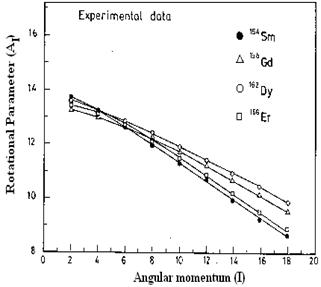Fig. (1). Experimental rotational constants (appropriate to the transitions) plotted against angular momentum, I for 154Sm92, 158Gd94, 162Dy96, and 166Er98 nuclei.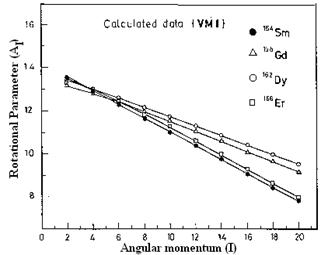Fig. (2). The calculated rotational constants plotted against angular momentum, I for the same nuclei in Fig. (1).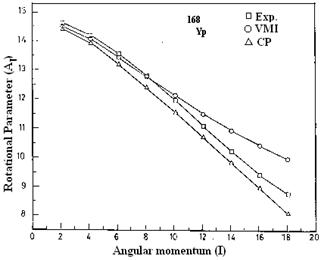Fig. (3). Experimental and calculated rotational constants plotted against angular momentum, I for 168Yb98 nucleus.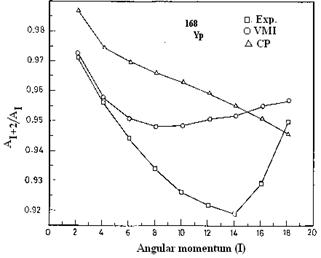Fig. (4). Ratio of successive experimental and calculated rotational constants, AIt2/AI, plotted against angular momentum, I for 168Yb98, nucleus.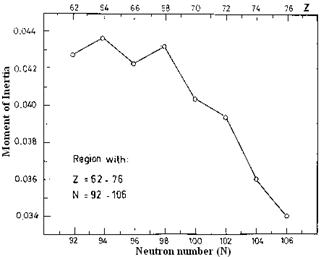Fig. (5). The inertial parameter for the ground state band plotted against neutron number in the region with N= 92-106 and Z=62-76.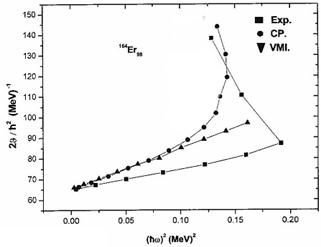Fig. (6). The observed moments of inertiaas a function of the square of the angular velocity (ħω)2 showing experimental and theoretical curves for 164Er. The figure shows the predicted value of the critical spin at which back bending is expected from the calculations.

From cubic polynomial (CP) formula the following expressions are derived by using a simple energy expression E(I)=aI+bI2+cI3 in equations 4 and 5 ,then

2I / ħ2 = (2I+1)[ a+2bI+3cI2 ]-1        (6)

And

(ħω)2 = [(I2+I+1)/(I2+I+¼)] [a+2bI+3cI2]2   (7)

The calculations yield positive a and b and negative c for all the nuclei studied. For high spin values the first factor in equation (7) is practically unity, and2 is seen to reach its maximum for the spin value Im = b / 3| c | . Since the spins of interest are only even integers we designate the even integer next nearest to Im as the critical spin Ic such that2 (Ic)2 (Ic-2). Calculations show that Ic value ( Ic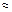20ħ) agree reasonable well with those experimentally observed so far ( Ic16ħ) .

3. Theoretical Calculations and Discussion

In the present calculations for all studied nuclei with neutron number N=92-98 (from Sm to Er nuclei), the ground state of several neutron deficient Sm, Gd nuclei, known experimentally to be essentially spherical, are therefore generally somewhat deformed (often oblate in the calculations). One typical features of some of the light rare earth nuclei is the fact that the ground state distortion, whether oblate as in 148Sm, 150Gd or prolate as in 154Sm92 ,158Gd94 ,162Dy96 ,166Er98 and 168Yb98 appears to contract in deformation parameter b with increasing I. The reason for the diminishment in b can be directly understood from an inspection of a figure exhibiting neutron orbitals as functions of b [ Ref.(1)]. For the heavy rare earth with N³ 100 the opposite effect is noticeable. Thus for 174Hf102, 178W104, and 182Os106 the effect of stretching is very apparent.

For the present calculations only transitions whose association with the ground-state rotational band is highly probable. From the transition energies, E (II-2), we define the rotational constant, AI as follows:

A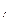= ħ2/2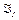= (E- E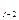) / (4I-2)     (8)

whererepresents the moment of inertia appropriate to the transition. Figures 1 and 2 show the analysis of the experimental and calculated values of the rotational parameter AI in terms of the angular momentum I for the nuclei studied. For comparison Fig. (2) included the result from a calculation of the variable moment of inertia VMI approach (which is one of the successful phenomenological models for even-even nuclei) in a detailed analysis of rotational bands in such nuclei. At low spin, the general features of this plot are well known :(1) a regular decrease in AI with increasing spin, and (2) smaller slopes (more perfect rotors) associated with lower AI values. The similarity in rotational properties of all these isotopes at higher spins is very pronounced.

The points in all cases are, or become with increasing spin, quite linear with a common limiting slope of 0.2 or0.3% decrease in AI per state. It can be ruled out that at still higher spins the AI values will diverge (Fig. 1, 2 and 3) again; however, the most tentative data at the highest spins rather suggests that they may converge to a single group. Thus from the lowest to the highest spins observed, the lighter rare-earth nuclei such as 154Sm and 158Gd seem to have rotational constant 3-6% lower than others in the region studied here (96-106 neutrons inclusive). A possible explanation is that this is due to reduction of the pairing correlation due to the energy gap in the Nilsson diagram (25). This effect seems to be reproduced in Nilsson calculations of the moment of inertia based on the pairing model (25). The very nearly identical behavior of the moments of inertia observed at high spin values for the nuclei studied suggests that a very general property of rotating nuclei must be involved. The average change in moment of inertia with spin observed in this study is about a factor of two, which indicates that an attempt to explain this should avoid using perturbation theory.

Table (1). Experimental and calculated rotational parameter values with the corresponding angular momentum in 154Sm 92 , 158Gd94 , 162 Dy96 and 166Er 98 nuclei.

 Nucleus 154Sm 158Gd 162Dy 166Er AI I Exp. Cal. Exp. Cal. Exp. Cal. Exp. Cal. 2 13.67 13.51 13.25 13.12 13.64 13.49 13.43 13.38 4 13.21 12.90 12.99 12.82 13.12 13.06 13.17 13.02 6 12.61 12.28 12.62 12.41 12.86 12.62 12.75 12.46 8 11.97 11.64 12.20 11.97 12.34 12.18 12.16 11.86 10 11.32 11.00 11.74 11.51 11.94 11.74 11.55 11.28 12 10.72 10.36 11.22 11.05 11.43 11.30 10.80 10.58 14 10.12 9.72 10.88 10.58 10.96 10.86 10.24 9.94 16 9.48 9.08 10.36 10.06 10.46 10.42 9.58 9.28 18 8.74 8.44 9.84 9.64 9.90 9.98 8.93 8.63

A comparison of the experimental data with the calculations by using the variable moment of inertia (VMI) and Cubic polynomial formula fit (CP) for the 168Yb98 nuclei is shown in Fig. (4). this case 168Yb98, represents one of the best fits of our nuclei. The ordinate here is the ratio AI+2/AI,

AI+2/AI = [E (I+2I) / 4I+6]/ [E (II-2) /4I-2]   (9)

which is related to the slope of Fig. (1), and is used primarily because it gives a plot which is very sensitive to the transition energies. A least-square fit has been used to calculate the energy spectra to the ground state bands. For all studied even – even nuclei, the model parameters a, b and c can be determined from fitting the three energy levels E2, E4 and E6. It can be seen that the quality of fit, with an error around ± 0.3% between the calculated and experimental energy levels. The moment of inertia obtained for the studied nuclei are compared. The value of the inertial parameter tends to fluctuation until N= 98 and after that tend to decrease smoothly with neutron number as shown in fig. (5). Again there is a systematic similarity between the nuclei under investigation: although the interactions in these nuclei are stronger, both interactions initially decrease with neutron. These comparisons strongly suggestive of an underlying structural similarity of the intrinsic states in these nuclei.

Table (2). Experimental and calculated ratios of successive rotational constants with the corresponding angular momentum in 168Yb98 nucleus .

 I AI (Exp) AI (VMI) AI (CP) AI t 2 (Exp) AI t 2 (VMI) AIt2 (CP)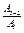(Exp.)(VMI)(CP) 2 14.62 14.48 14.457 14.201 14.080 14.267 0.9713 0.97237 0.9869 4 14.20 14.08 13.966 13.579 13.484 13.610 0.95626 0.9577 0.9745 6 13.58 13.48 13.227 12.825 12.820 12.830 00.9444 0.95104 0.96998 8 12.82 12.82 12.417 11.980 12.160 12.000 0.93447 0.94852 0.9664 10 11.98 12.16 11.574 11.100 11.540 11.150 0.92654 0.94901 0.9633 12 11.10 11.54 10.720 10.240 10.980 10.290 0.92252 0.95147 0.95988 14 10.24 10.98 9.854 9.420 10.960 9.420 0.91992 0.95264 0.9559 16 9.43 10.46 8.983 8.770 10.000 8.550 00.9300 0.95603 0.95179 18 8.77 10.00 8.100 8.340 9.580 7.670 0.95079 0.9580 0.9469

4. Conclusion

The prolate and oblate configurations in N@92-106 nuclei interact strongly perturbing states. Levels perturbed in their way must contain contributions from both prolate and oblate wave functions. This is due to level mixing and shape coexistence previously seen in much lighter rare earth nuclei such as 154Sm and 158Gd. Also, the ground-state rotational band members for several nuclei have been identified and provide a good test of various models for rotational bands. An impressive agreement is observed with a variable moment of inertia and cubic polynomial calculation, where the rotational parameters are related to I, give equally good results.

References

1. A. Bohr and B.R. Mottelson ; Phys. Scripta 10A (1974) 13.
2. R. Bengtsson ; S.E. Larsson ; G. Leander ; P. Möller ; S.G. Nilsson ; S. Äberg and Z. Szymanski, Phys, Lett. 57B (1975) 310.
3. K. Neergaard and V.V. Pashkevich ; Phys. Lett. 59B (1975) 218.
4. G. Andersson ; S.E. Larsson ; G. Leander ; P. Möller ; S.G. Nilsson ; I. Ragnarsson and S. Äberg ; R. Bengtsson and J. Dudek ; B. Nerlo-Pomorska, K. Pomorski and Z. Szymanski ; Nucl. Phys. A268 (1976) 205.
5. N.A. Mansour and A.M. Diab ; Indian J. Phys.77A(3),289 (2003) and references there in.
6. William ; M.C. Latchie and W. Darcey and J.E. Kitching ; Nucl. Phys. A159 (1970) 615.
7. G. Løvhøiden ; T.F. Thorsteinsen ; E. Andersen and M.F. Kiziltan ; D.G. Burkem ; Nucl. Phys. A494 (1989) 157.
8. H.H. Pitz ; U.E.P. Berg ; R.D. Hell ; U.Kneissl and R. Stock ; C. Wesslborg and P. Von Brentana ; Nucl. Phys. A492 (1989) 411.
9. L. Henden ; L. Bergholt ; M. Guttormsen ; J. Rekstad ; T.S. Tveter ; Nucl. Phys. A589 (1995) 249.
10. J. Rekstad ; A. Atac ; M. Guttormsen ; T. Ramsdy ; J.B. Olsen and F. Ingebretsen ; T.F. Thorsteinsen ; G. Løvhøiden and T. Rødland ; Nucl. Phys. A470 (1987) 397.
11. J.P. Lestone ; J.R. Leigh ; J.O. Newton ; D.J. Hinde ; J.X. Wei ; J.X. Chen ; S. Elfström ; M. Zielinska-Pfabe ; Nucl. Phys. A559 (1993) 277.
12. C.A. Fields and K.H. Hickes and R.J. Peterson ; Nucl. Phys. A440 (1985) 301.
13. J.C. Lisle; D. Clarkc ; R.Champman ; F. Khazaie ; and J.N. Mo ; H. Hübel ; W. Schmitz and K. Thiene ; J.D. Garrett ; G.B. Hagemann ; B. Herskind and K. Schiffer ; Nucl. Phys. A520 (1990) 451, and procedings of the conference on nuclear structure in the nineties Oak Ridge. Tennesse, April 23, (1990).
14. P.M. Walker ; G.B. Hagamann; J. Pedersen; G. Sletten ; D. Howe; M.A. Riley ; B.M. Nyako; J.F. Sharpey-Schafer; J.C. Lisle; E. Paul; Daresbury Annual report (1983/84) 43.
15. P. M. Walker ; G. Sletten ; N.L. Gjorup ; J. Borggreen ; B. Fabricius ; A. Holm ; J. Pederson ; M.A. Bentley ; D. Howe ; J.W. Reberts ; J.F. Sharpey-Schafer ; Daresbury Annual report (1989/90) 66.
16. A. Krämer-Flecken ; T. Morek ; R.M. Lieder ; W. Gast ; G. Hebbinghaus ; H.M. Jäger ; W. Urban ; Annual report, Jülich GmbH Institute Für kernphysik (1988) 36.
17. H. Schnare ; A. Krämer-Fleckem ; D. Balabonski ; W. Gast ; G. Hekbinghaus ; R.M. Lieder ; M.A. Bontley ; D. Howe ; A.R. Mokhtar ; J.D. Morrison ; J.F. Sharpey-Schafer ; P.M. Walker ; Annual report, Jülich GmbH Institute Für kernphysik (1988) 43.
18. A. Ansari ; M.Oi ; N. Onishi ; T. Horibata ; Nucl. Phys. A654 (1999) 558.
19. Takatoshi Horibata; M.Oi ; N. Onishi; A. Ansari ; Nucl.Phys. A646(1999) 277.
20. Takatoshi Horibata ; N. Onishi ; Nucl. Phys. A596 (1996) 251.
21. T. Kutsarova ; R.M. Lieder ; H. Schnare ; G. Hebbinghaus ; D. Balobanski ; W. Gast ; A. Krämer-Flecken ; M.A. Bentley ; P. Fallon ; D. Howe ; A.R. Mokhtar ; J.F. Sharpey-Schafer ; P. Walker ; P. Chowdhury ; B. Fabricius ; G. Sletten ; S. Frauendorf ; Nucl. Phys. A 587 (1995) 111.
22. T. Kibédi ; G.D.Dracoulis; A. P. Byrne ; P.M. Davidson ; S. Kuyucak ; Nucl. Phys. A567 (1994) 183.
23. N.A. Mansour and A.M. Diab ; Indian J. Phys.77A(4), 377 (2003).
24. Goldhaber A.S and Scharff-Goldhaber G. ; Phys.Rev.C17,1171 (1978).
25. S.G. Nilsson and O.Prior, Kgl. Danske Videnskab.Selskab; Mat.-Fys.Medd. 32, No.16 (1991).

 Contents 1. 2. 3. 4.
Article ToolsAbstractPDF(256K)